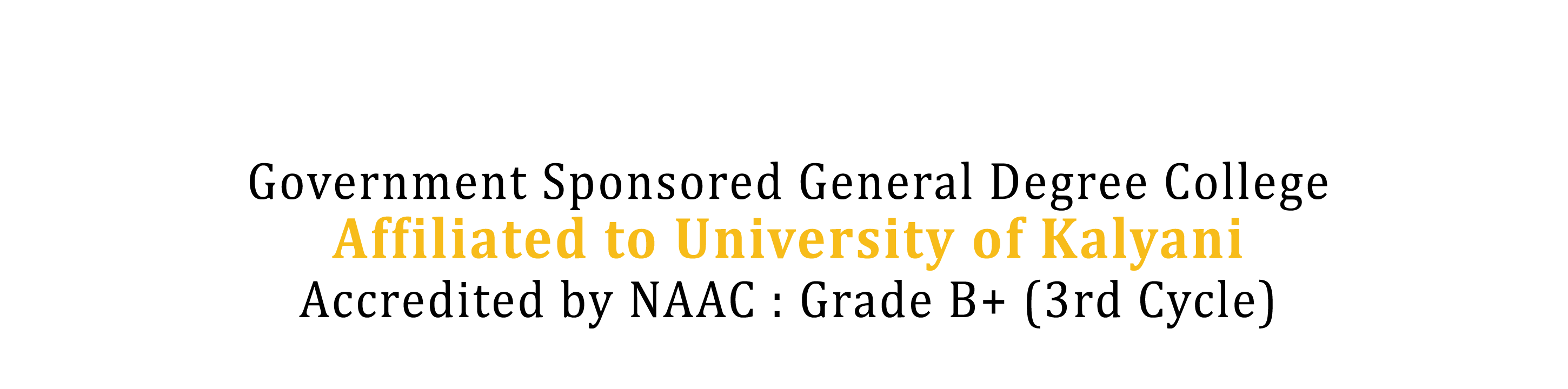Department Home
Department of Mathematics
Outcome of the Department

COURSES OUTCOME:

• Courses Outcome 1: Working Knowledge
Students will demonstrate a working knowledge of selected topics from calculus, linear algebra and distribution of other branches of mathematics.
• Courses Outcome 2: Proofs & Arguments
Students will demonstrate the ability to determine the validity of a given argument, and be able to construct mathematical proofs independently.
• Courses Outcome 3: Algorithms
Students will demonstrate understanding of the mathematical basis of common algorithms, and the ability to calculate accurately and efficiently.
• Courses Outcome 4: Problem Solving
Students will demonstrate the ability to solve problems, including applications outside of mathematics, by means of intuition, creativity, guessing and the experience gained through the study of particular examples and mathematical models. Students will demonstrate the ability to analyse data and draw appropriate mathematical & statistical conclusions.
• Courses Outcome 5: Mathematical Communication
Students will demonstrate the ability to communicate mathematical ideas clearly. They will use correct mathematical terminology and proper mathematical notation.
• Courses Outcome 6: Employment skills
Students should attain the needed written and oral communication skills to translate their degree into a viable career path
• Courses Outcome 7: Ethical Leadership
Students will demonstrate the ability to think critically, research, and reason.
• Courses Outcome 8: Learning Teaching Techniques
Students will demonstrate the ability to effectively utilize a variety of teaching techniques and classroom strategies to positively influence student learning.
• Courses Outcome 9: Cultural Competence
Students will recognize and differentiate among diverse cultures through the history of mathematics.
Our Location